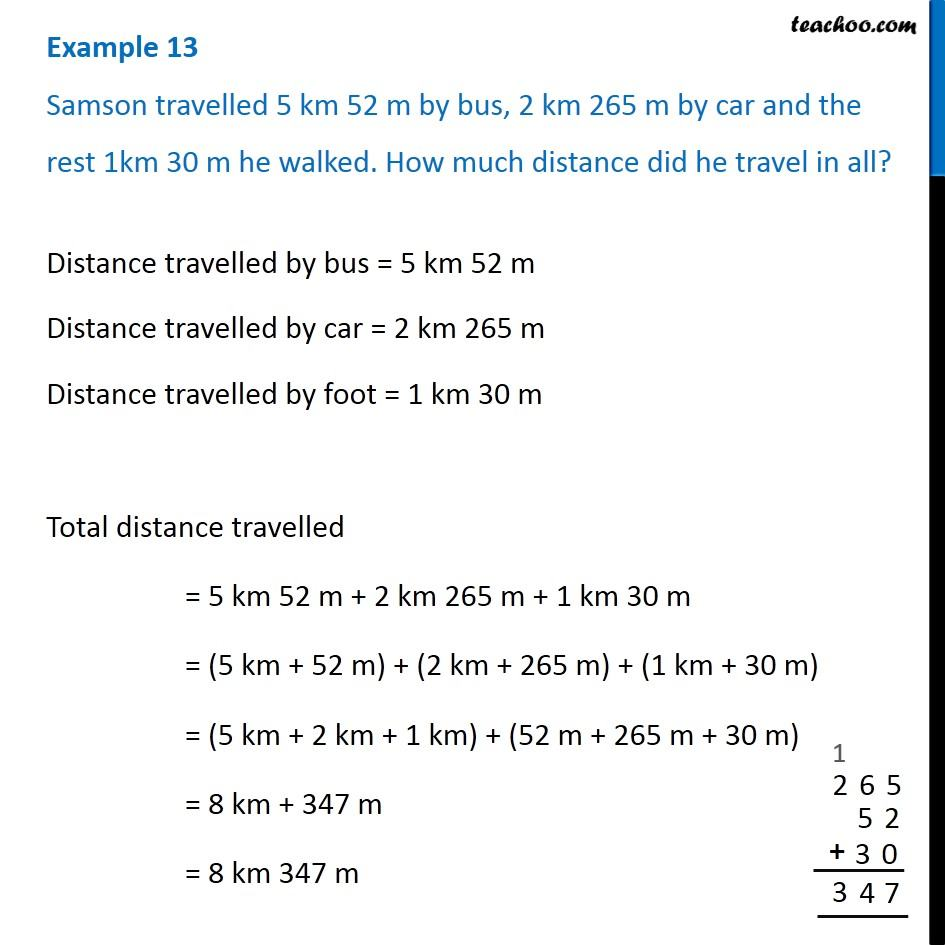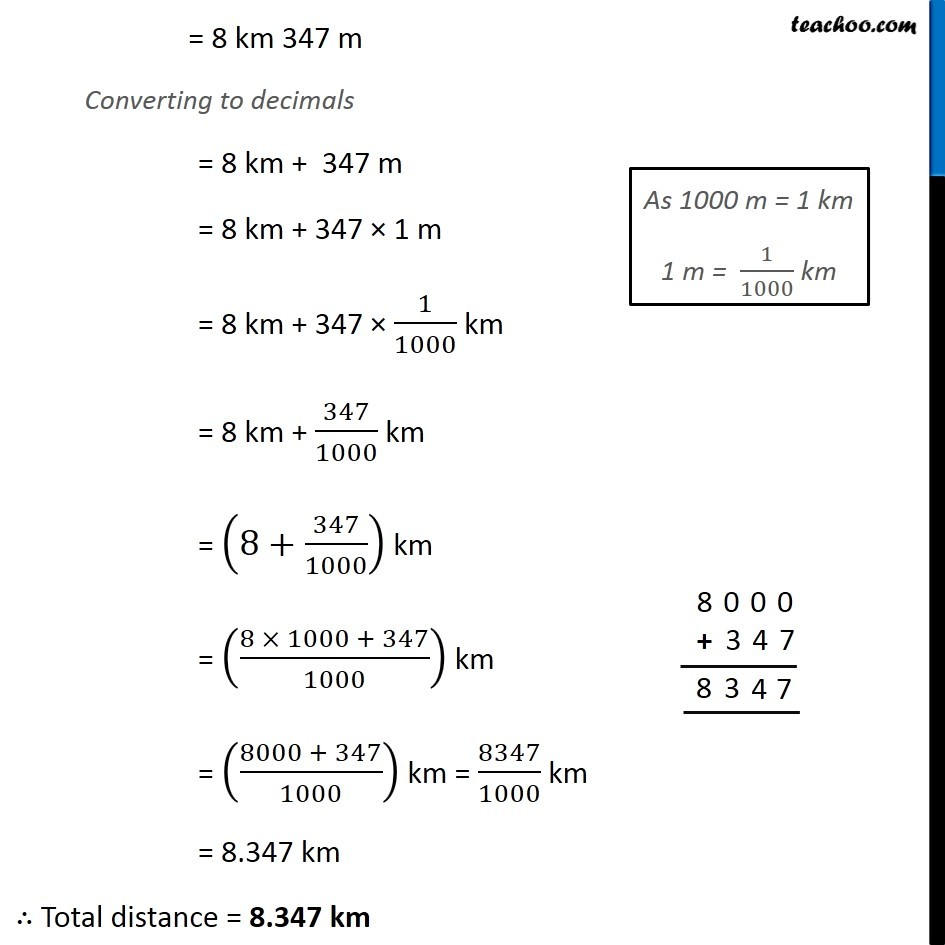Chapter 8 Class 6 Decimals
Concept wiseLearn in your speed, with individual attention - Teachoo Maths 1-on-1 Class

### Transcript

Example 3 Samson travelled 5 km 52 m by bus, 2 km 265 m by car and the rest 1km 30 m he walked. How much distance did he travel in all? Distance travelled by bus = 5 km 52 m Distance travelled by car = 2 km 265 m Distance travelled by foot = 1 km 30 m Total distance travelled = 5 km 52 m + 2 km 265 m + 1 km 30 m = (5 km + 52 m) + (2 km + 265 m) + (1 km + 30 m) = (5 km + 2 km + 1 km) + (52 m + 265 m + 30 m) = 8 km + 347 m = 8 km 347 m = 8 km 347 m Converting to decimals = 8 km + 347 m = 8 km + 347 × 1 m = 8 km + 347 × 1/1000 km = 8 km + 347/1000 km = (8+347/1000) km = ((8 × 1000 + 347)/1000) km = ((8000 + 347)/1000) km = 8347/1000 km = 8.347 km ∴ Total distance = 8.347 km